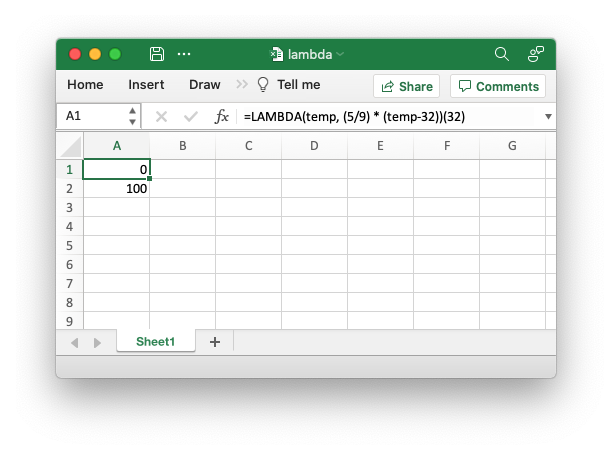# Example: Excel 365 LAMBDA() function#

This program is an example of using the new Excel `LAMBDA()` function. It demonstrates how to create a lambda function in Excel and also how to assign a name to it so that it can be called as a user defined function. This particular example converts from Fahrenheit to Celsius.

See the The Excel 365 LAMBDA() function section of the documentation for more details.```#######################################################################
#
# An example of using the new Excel LAMBDA() function with the XlsxWriter
# module.
#
# Copyright 2013-2023, John McNamara, jmcnamara@cpan.org
#
from xlsxwriter.workbook import Workbook

workbook = Workbook("lambda.xlsx")

# Write a Lambda function to convert Fahrenheit to Celsius to a cell.
#
# Note that the lambda function parameters must be prefixed with
# "_xlpm.". These prefixes won't show up in Excel.
worksheet.write("A1", "=LAMBDA(_xlpm.temp, (5/9) * (_xlpm.temp-32))(32)")

# Create the same formula (without an argument) as a defined name and use that
# to calculate a value.
#
# Note that the formula name is prefixed with "_xlfn." (this is normally
# converted automatically by write_formula() but isn't for defined names)
# and note that the lambda function parameters are prefixed with
# "_xlpm.". These prefixes won't show up in Excel.
workbook.define_name("ToCelsius", "=_xlfn.LAMBDA(_xlpm.temp, (5/9) * (_xlpm.temp-32))")

# The user defined name needs to be written explicitly as a dynamic array
# formula.
worksheet.write_dynamic_array_formula("A2", "=ToCelsius(212)")

workbook.close()
```